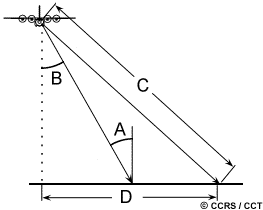# Vertical and horizontal displacement

Hi there,

1. I calculated the LOS displacement by following the SARedu tutorial. I wanna now to calculate the vertical and horizontal displacements. How to do that using SNAP if I have displacement calculated from two descending Sentinel-1A?
2. Is it there any way to separate the horizontal displacement into N-S and and E-W displacements?
3. Does the use of two VV or two VH polarization give correct (almost all the same) results for measuring the LOS displacement?

Regards

3 Likes

By combining LOS displacement values of both ascending and descending directions you can calculate the actual direction with the Pythagoras theorem.
But differentiating between horizontal and lateral movement is hard to do.

Thanks for responding,
Can you give me more detail about “Pythagoras theorem”, and how to apply it?

Regards

Usually vv is the better choice for InSAR, but it doesn’t make much difference. Compare which of both has the better choherence. Using both doesn’t make much sense.

Pythagoras may have been the wrong term, sorry. I just meant that you can add up displacement values of ascending (a) and descending (b) movement to improve your result regarding absolute © directions using vector maths:

Source

Sorry for disturbing you, can we get a benefit from incidence angle?

Cheers

1 Like

yes, different incidence angles affect the line of sight. Large displacement along a steep angle are of course more severe than large displacement values along a flat angle.

But note that the incidence angle is measured from the surface normalSource: http://www.nrcan.gc.ca/node/9341

Thanks a lot,
still it is not clear for me. I found this figure that explain the distribution of the displacement.

Moreover, I had read the tutorial no. 3102 of SARedu that they calculate the vertical displacement by using the cosine of incidence angle multiplied by the displacement. But I guess it type of simplify the problem.

Cheers

1 Like

Have you got the vertical displacement in SNAP software and how? Now I have got the displacement map, I wanna to transfer it to vertical displacement. Thanks.

Hi,
As I understand, and please Andreas correcting that, if there is some mistakes. You should have two results, ascending and descending . You must calculate the cosine of incidence angle multiplied by the LOS displacement for both ascending and descending. and finally take the average of the two results.

Cheers

Hi,
I am trying to get the average of displacemnt from the Ascending and Decending outputs, but I am having trouble to insert both of them in an equation in the Band Math.
Is there a way to be able to insert both of them to the Band Math or to merge them to one product in order to be able to insert them in the Band Math?
Thanks,

please have a look at these posts of mengdahl and the reference he gives:

Hi ABraun
Thank you for these useful links. I can find the displacement from ascending or descending unwrapped output Separately. I am asking whether there is a way to be insert two file in the Band Math in the Snap? there may be an alternative.
In that case you can also use Incidence angle file instead of just using a number. It will be more precise since the angle itself vary from the east to the west of scene.

about the second, the incidence angle for each pixel is usually available in the tie-point-grids can be used as a variable in the band maths.
For the first, if you want to use the rasters of two products in the band maths you first have to stack them into one product.

1 Like

I’ve computed vertical displacement, but how can do that for horizontal ones?

Sincerely,

1 Like

Hello everyone,
i’m trying to compute an estimation of vertical and horizontal displacement from the LOS by using only the incidence angle. Im using images from one orbit.
v = los · cos (incidence angle)
h = los · sin (incidence angle)

However, I’m sure that in this way I’m introducing an error in comparison to the formulas that use images from two orbits.

Can someone, even discursively, indicate me the errors that I introduced and the implicit hypothesis assumed by using
v = los · cos (incidence angle)
h = los · sin (incidence angle)
relations?